##### PostgreSQL SUM() Function With Practical Examples

PostgreSQL provides a built-in SUM() function that is used to perform the addition on a set of values. Postgres allows us to compute the sum of distinct values using an additional option/operator, DISTINCT. While performing addition, the SUM() function skips the “NULL” values.

Let’s learn the working of the SUM() function through Practical examples.

How to Use SUM() Function in Postgres?

The below snippet illustrates the syntax of the SUM() function:

`SUM(DISTINCT exp | col_name);`

Here, “exp” represents an expression while col_name represents the column name. In the SUM() function, either you can specify an expression to calculate its sum, or you can specify a column name on which you want to perform the addition.

Example #1: How to Use SUM() Function on Table’s Data?

Let’s say we have a table bike_details. We will execute the SELECT query to fetch all the records of the bike_details table:

`SELECT * FROM bike_details;`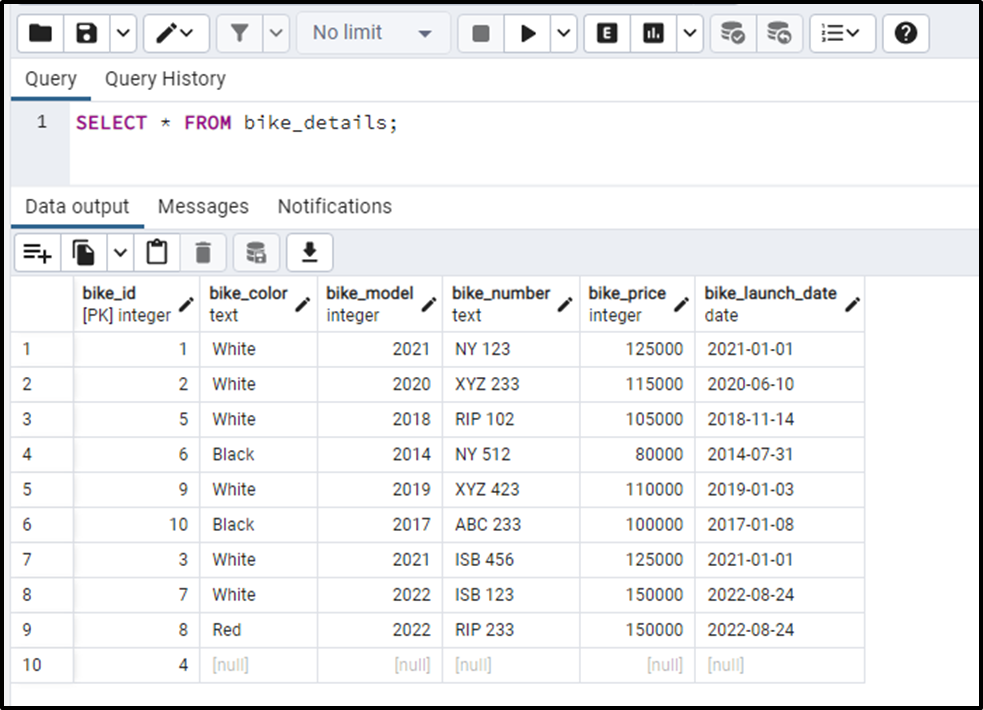Let’s perform addition on the bike_price column using the SUM() Function:

```SELECT SUM(bike_price) AS total_price
FROM bike_details;```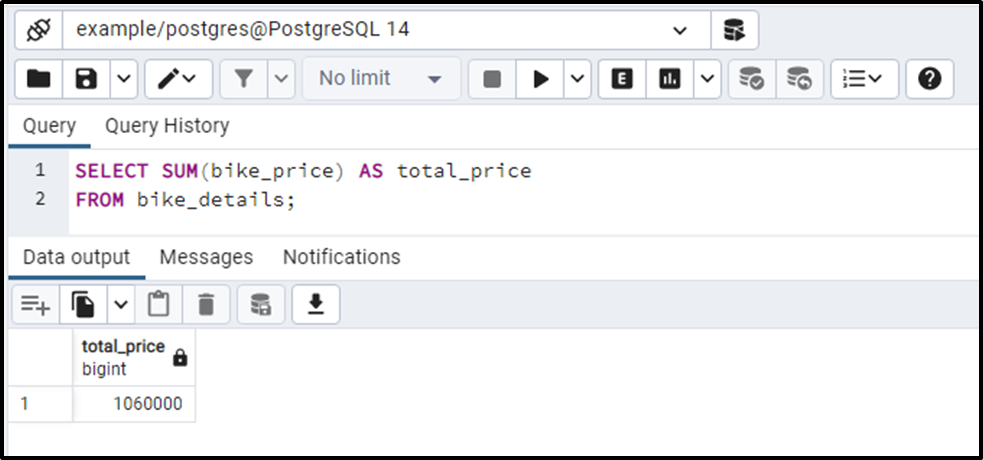The output authenticates that the SUM() function returns the sum of the bike_price column.

Example #2: How to Calculate the Sum of Distinct Values Using the SUM() Function?

Specify the DISTINCT option before the column’s name to perform the addition on the distinct values:

```SELECT SUM(DISTINCT bike_price) AS total_price
FROM bike_details;```

Here is what you will get on successful execution of the above-given query: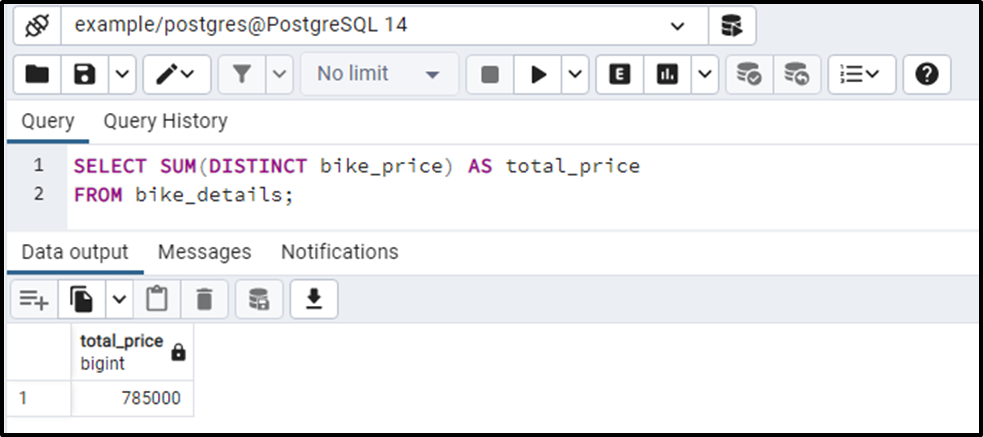This time, the SUM() function returned the sum of distinct values.

Example #3: How to Use the SUM() Function to Compute the Sum of Groups?

Run the following query to perform the addition on the bike_price column based on the groups. Let’s utilize the GROUP BY clause to group the bikes having the same model:

```SELECT
bike_model,
SUM (bike_price) AS total_price
FROM bike_details
GROUP BY bike_model;```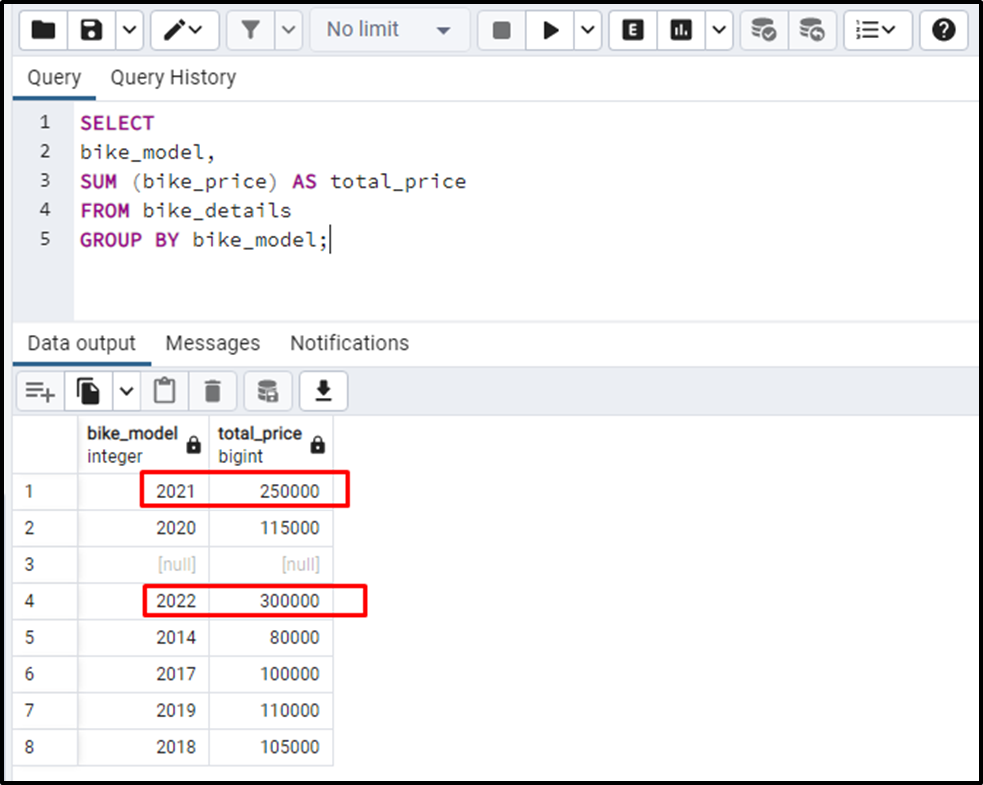From the output, you can observe that this time the SUM() function returned the sum in the form of groups.

Example #4: How to Use SUM() Function With HAVING Clause in Postgres?

Let’s perform the addition on the bike_price column based on a specific condition, i.e., bike price >= 115,000. To do that, we will use the Postgres HAVING Clause:

```SELECT
bike_model,
SUM (bike_price) AS total_price
FROM bike_details
GROUP BY bike_model
HAVING SUM(bike_price) > 115000;```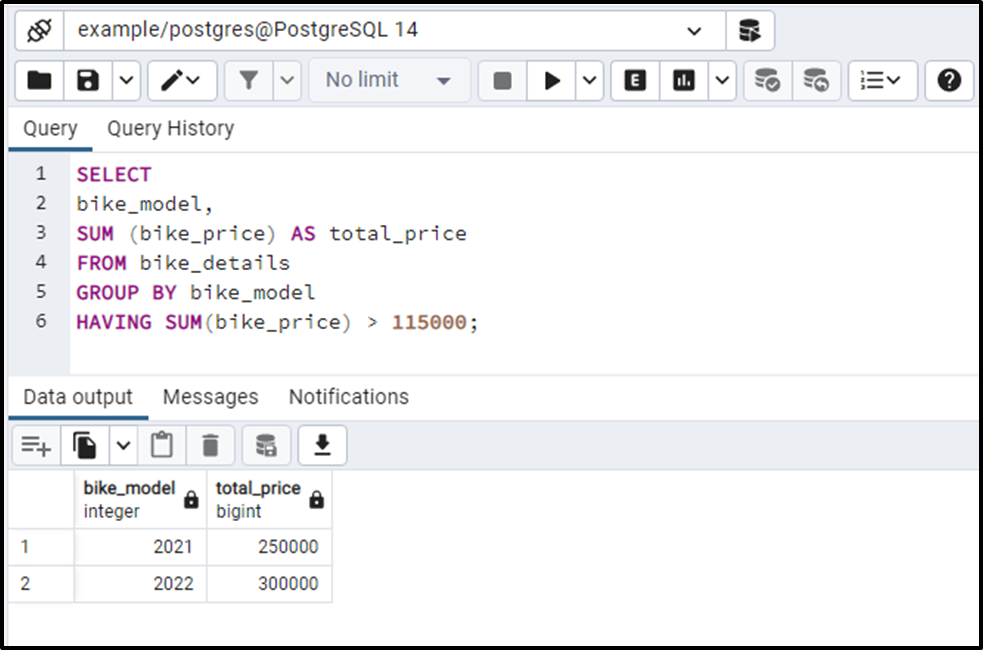In this example, we utilized the SUM() function to calculate the sum of the bike_price column. Next, we utilized the GROUP BY clause to group the bikes based on their model and the HAVING clause to specify a condition.

Example #4: How to Use SUM() Function on An Expression in Postgres?

We modified the bike_details table and added a new column named “price_change”. The updated table will look like this:

`SELECT * FROM bike_details;`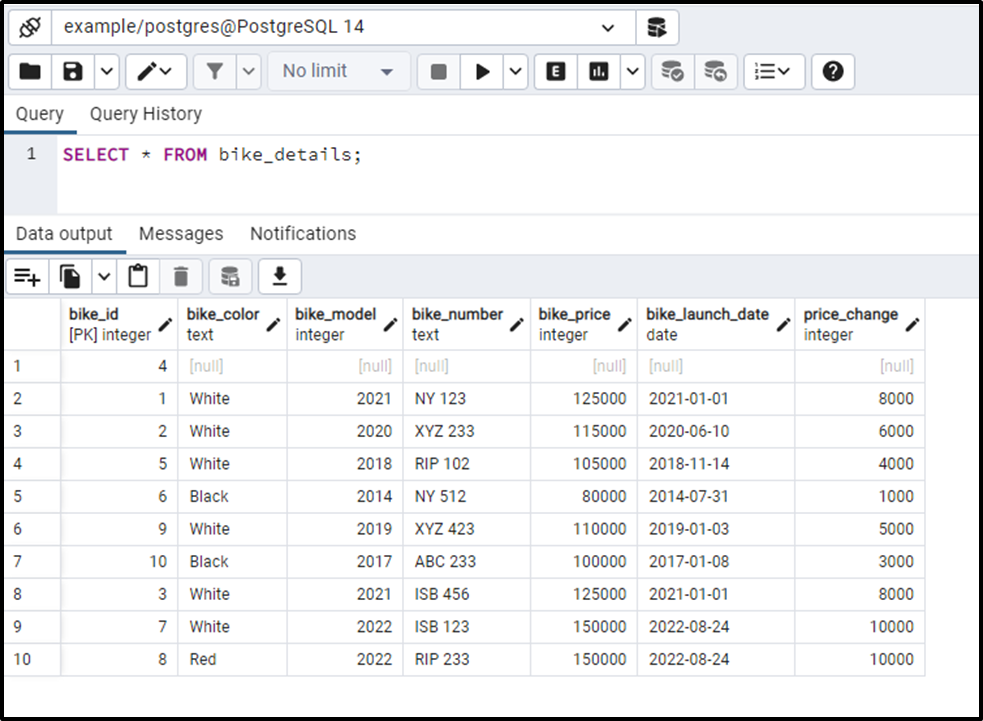Let’s specify an expression within the SUM() function and see how the SUM() function work:

```SELECT bike_model, SUM(DISTINCT bike_price + price_change)
FROM bike_details
GROUP BY bike_model;```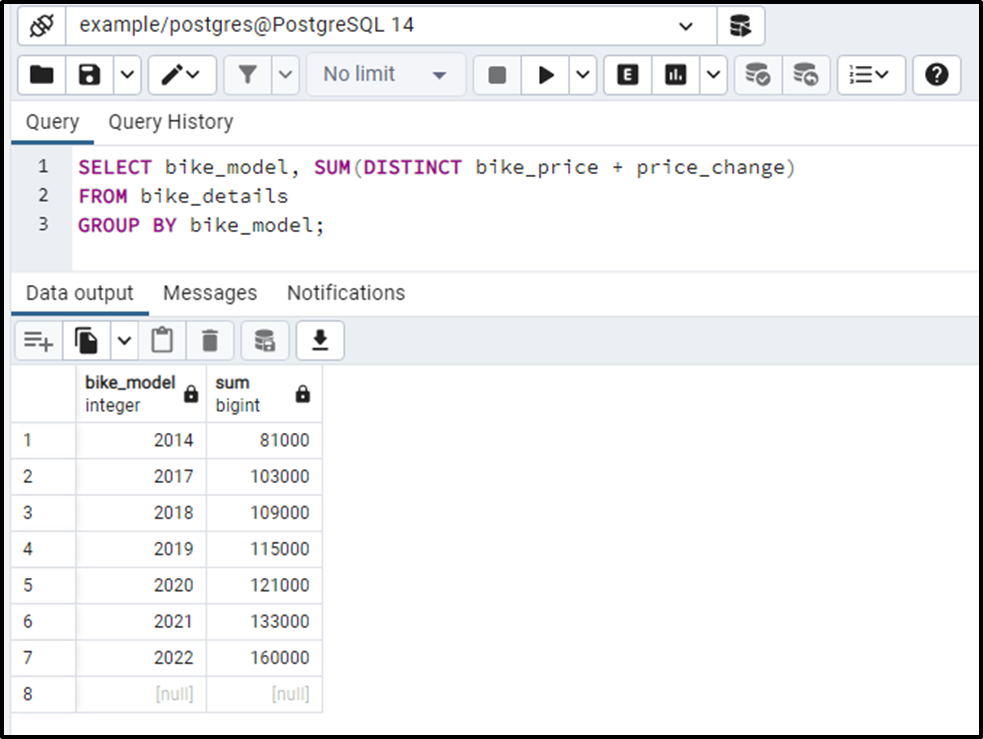In this example, we perform the addition on the bike_price and price_change column. We utilized the group by clause to show the updated price of each model.

This way, you can use the SUM() function to perform the addition in PostgreSQL.

Conclusion

PostgreSQL offers an in-built function named SUM() that is used to perform the addition on a set of values. Postgres allows you to compute the sum of distinct values using an additional option/keyword, DISTINCT. This write-up considered different scenarios to demonstrate the working of the SUM() function in PostgreSQL.